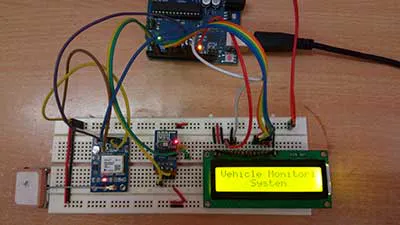# ESP8266 and Arduino Based IoT Vehicle Tracking System

This Arduino based Vehicle Tracking Device is an IoT device built by interfacing Neo 6M GPS module, ESP8266 Modem and character LCD to the A

IntermediateFull instructions provided3,238## Schematics

### Arduino and ESP8266 based IoT Vehicle Tracking Device Circuit## Code

### Source Code For ESP8266 and Arduino Based IoT Vehicle Tracking System

C/C++
```//Program to

#include<SoftwareSerial.h>

#include<LiquidCrystal.h>

SoftwareSerial Serial1(2,3);     //make RX arduino line is pin 2, make TX arduino line is pin 3.

SoftwareSerial gps(10,11);

LiquidCrystal lcd(13,12,9,8,7,6);

boolean No_IP=false;

String IP="";

String webpage="";

int i=0,k=0;

int  gps_status=0;

String place="<p>1. Name: [ENTER UR NAME] </p>";

String num = "<p>2. Vehicle No: IND001</p>";

String cordinat="<p>Coordinates:</p>";

String latitude="";

String logitude="";

String gpsString="";

char *test="\$GPGGA";

void setup()

{

Serial1.begin(9600);

Serial.begin(9600);

lcd.begin(16,2);

lcd.print("Vehicle Monitoring");

lcd.setCursor(0,1);

lcd.print("     System     ");

delay(2000);

lcd.clear();

lcd.print("Connecting....");

delay(1000);

connect_wifi("AT",1000);

connect_wifi("AT+CWMODE=3",1000);

connect_wifi("AT+CWQAP",1000);

connect_wifi("AT+RST",5000);

FindIP(5000);

if(!No_IP)

{

Serial.println("Connecting Wifi....");

connect_wifi("AT+CWJAP=\"[ENTER UR SSID NAME] \",\"[ENTER THE PASSWORD]\"",7000);

}

else

{

}

Serial.println("Wifi Connected");

lcd.clear();

lcd.print("WIFI Connected");

delay(2000);

lcd.clear();

lcd.setCursor(5,0);

lcd.print("IP");

lcd.setCursor(3,1);

delay(2000);

connect_wifi("AT+CIPMUX=1",100);

connect_wifi("AT+CIPSERVER=1,80",100);

Serial1.end();

lcd.clear();

lcd.print("   GPS DATA");

delay(2000);

gps.begin(9600);

get_gps();

coordinate_display();

gps.end();

Serial1.begin(9600);

}

void loop()

{

k=0;

while(k<1000)

{

k++;

while(Serial1.available())

{

if(Serial1.find("0,CONNECT"))

{

Serial1.end();

gps.begin(9600);

get_gps();

gps.end();

Serial1.begin(9600);

Serial1.flush();

Serial.println("Start Printing");

Send();

coordinate_display();

Serial.println("Done Printing");

delay(5000);

lcd.clear();

delay(1000);

k=1200;

break;

}

}

delay(1);

}

}

void FindIP(int t1)

{

int t2=millis();

while(t2+t1>millis())

{

while(Serial1.available()>0)

{

if(Serial1.find("WIFI GOT IP"))

{

No_IP=true;

}

}

}

}

{

IP="";

char ch=0;

while(1)

{

Serial1.println("AT+CIFSR");

while(Serial1.available()>0)

{

if(Serial1.find("STAIP,"))

{

delay(1000);

while(Serial1.available()>0)

{

if(ch=='+')

break;

IP+=ch;

}

}

if(ch=='+')

break;

}

if(ch=='+')

break;

delay(1000);

}

lcd.clear();

lcd.print(IP);

lcd.setCursor(0,1);

lcd.print("Port: 80");

Serial.print(IP);

Serial.print("Port:");

Serial.println(80);

delay(1000);

}

void connect_wifi(String cmd, int t)

{

int temp=0,i=0;

while(1)

{

Serial.println(cmd);

Serial1.println(cmd);

while(Serial1.available()>0)

{

if(Serial1.find("OK"))

{

i=8;

}

}

delay(t);

if(i>5)

break;

i++;

}

if(i==8)

{

Serial.println("OK");

}

else

{

Serial.println("Error");

}

delay(1000);

}

void gps_Function()

{

gpsString="";

while(1)

{

while (gps.available()>0)                //Serial incoming data from GPS

{

gpsString+= inChar;                    //store incoming data from GPS to temporary string str[]

i++;

if (i < 7)

{

if(gpsString[i-1] != test[i-1])       //check for right string

{

i=0;

gpsString="";

}

}

if(inChar=='\r')

{

if(i>65)

{

gps_status=1;

break;

}

else

{

i=0;

}

}

}

if(gps_status)

break;

}

}

void get_gps()

{

gps_status=0;

int x=0;

while(gps_status==0)

{

gps_Function();

int str_lenth=i;

latitude="";

logitude="";

coordinate2dec();

i=0;x=0;

str_lenth=0;

}

}

void coordinate_display()

{

lcd.clear();

lcd.print("LATITUTE:");

lcd.print(latitude);

lcd.setCursor(0,1);

lcd.print("LONGITUTE:");

lcd.print(logitude);

Serial.print("Latitude:");

Serial.println(latitude);

Serial.print("Longitude:");

Serial.println(logitude);

}

void coordinate2dec()

{

String lat_degree="";

for(i=18;i<20;i++)          //extract latitude from string

lat_degree+=gpsString[i];

String lat_minut="";

for(i=20;i<28;i++)

lat_minut+=gpsString[i];

String long_degree="";

for(i=30;i<33;i++)          //extract longitude from string

long_degree+=gpsString[i];

String long_minut="";

for(i=33;i<41;i++)

long_minut+=gpsString[i];

float minut= lat_minut.toFloat();

minut=minut/60;

float degree=lat_degree.toFloat();

latitude=degree+minut;

minut= long_minut.toFloat();

minut=minut/60;

degree=long_degree.toFloat();

logitude=degree+minut;

}

void Send()

{

webpage = "<h1>Welcome to Engineers Garage</h1><body bgcolor=f0f0f0>";

webpage+=place;

webpage+=num;

webpage+=cordinat;

webpage+="<p>Latitude:";

webpage+=latitude;

webpage+="</p>";

webpage+="<p>Longitude:";

webpage+=logitude;

webpage+="</p>";

webpage+=latitude;

webpage+='+';              //28.612953, 77.231545   //28.612953,77.2293563

webpage+=logitude;

webdata();

webpage="";

while(1)

{

Serial.println("AT+CIPCLOSE=0");

Serial1.println("AT+CIPCLOSE=0");

while(Serial1.available())

{

if(Serial1.find("0,CLOSE"))

{

return;

}

}

delay(500);

i++;

if(i>5)

{

i=0;

}

if(i==0)

break;

}

}

void webdata()

{

i=0;

while(1)

{

unsigned int l=webpage.length();

Serial1.print("AT+CIPSEND=0,");

Serial1.println(l+2);

Serial.println(l+2);

Serial.println(webpage);

Serial1.println(webpage);

while(Serial1.available())

{

if(Serial1.find("OK"))

{

return;

}

}

i++;

if(i>5)

i=0;

if(i==0)

break;

delay(200);

}

}
```

## Credits

### TechnicalEngineer

4 projects • 29 followers